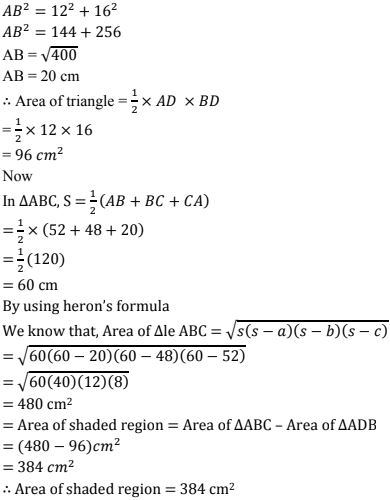# Chapter 12 Heron's Formula RD Sharma Solutions Exercise 12.1 Class 9 Maths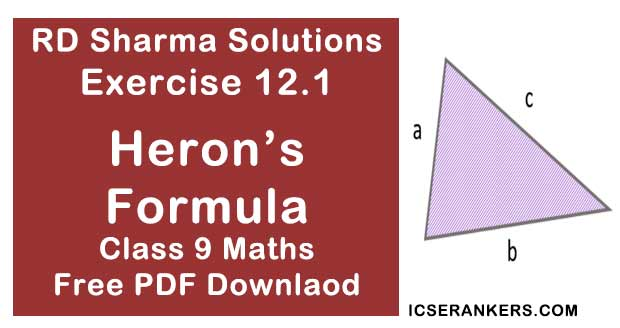Chapter Name RD Sharma Chapter 12 Heron's Formula Exercise 12.1 Book Name RD Sharma Mathematics for Class 10 Other Exercises Exercise 12.2 Related Study NCERT Solutions for Class 10 Maths

### Exercise 12.1 Solutions

1. Find the area of a triangle whose sides are respectively 150 cm, 120 cm and 200 cm.

Solution

The triangle whose sides are
a = 150 cm
b = 120 cm
c = 200 cm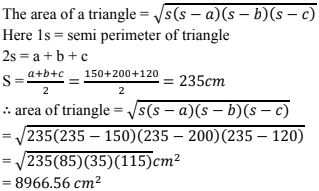2. Find the area of a triangle whose sides are  9 cm, 12 cm and  15cm.

Solution

The triangle whose sides are a = 9 cm, b = 12cm and c = 15 cm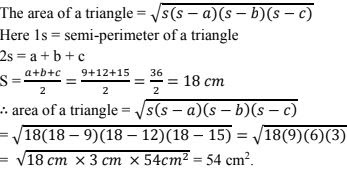3. Find the area of a triangle two sides of which are 18 cm and 10 cm and the perimeter is 42 cm.

Solution

21√11 cm2

4. In a ΔABC, AB = 15 cm, BC = 13 cm and AC = 14 cm. Find the area of ΔABC and hence its altitude on AC.
Solution
The triangle sides are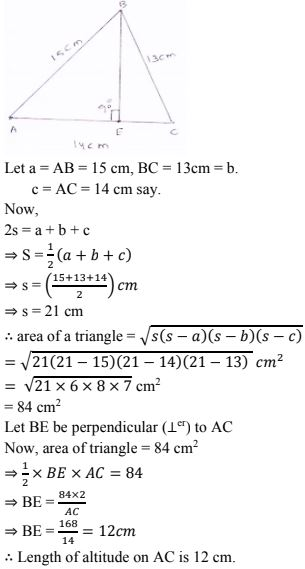5. The perimeter of a triangular field is  540m and its sides are in the ratio 25 : 17 :12. Find the area of the triangle.
Solution
The sides of a triangle are in the ratio 25: 17 : 12
Let the sides of a triangle are a = 25x, b = 17x and c = 12x say.
Perimeter = 25 = a + b + c = 540 cm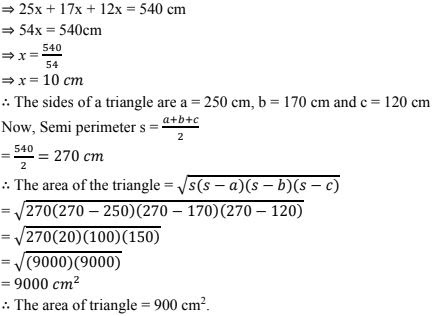6. The perimeter of a triangle is 300 m. If its sides are in the ratio 3 : 5 : 7. Find the area of the triangle.
Solution
Given that
The perimeter of a triangle = 300 m
The sides of a triangle in the ratio 3 : 5 : 7
Let 3x, 5x, 7x  be the sides of the triangle
Perimeter ⇒ 2x = a + b + c
⇒ 3x + 5x +7x = 300
⇒ 15x = 300
⇒ x =20m
The triangle sides are a = 3x
= 3(20)m = 60 m
b = 5x = 5(20) m = 100 m
c = 7x = 140 m
Semi perimeter s = (a + b + c)/2
= 300/2 = 150m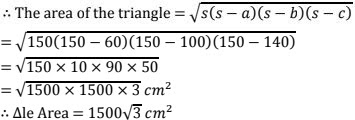7. The perimeter of a triangular field is 240 dm. If two of its sides are 78 dm and 50dm, find the length of the perpendicular on the side of length 50 dm from the opposite vertex.
Solution
ABC be the triangle, Here a = 78 dm = AB,
BC = b = 50 dm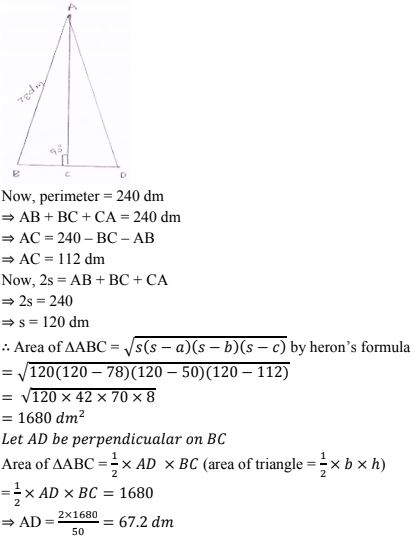8. A triangle has sides 35 cm, 54 cm and 61 cm long. Find its area. Also, find the smallest of its altitudes.
Solution
The sides of a triangle are a  = 35 cm, b = 54 cm and c = 61 cm
Now, perimeter a + b + c = 25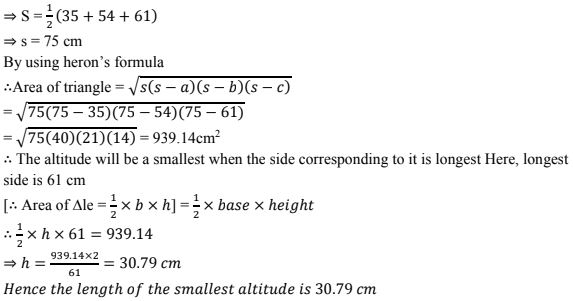9. The lengths of the sides of a triangle are in the ratio 3 : 4 : 5 and its perimeter is  144 cm. Find the area of the triangle and the height corresponding to the longest side.
Solution
Let the sides of a triangle are 3x, 4x and 5x.
Now, a = 3x, b = 4x and c = 5x
The perimeter 2s = 144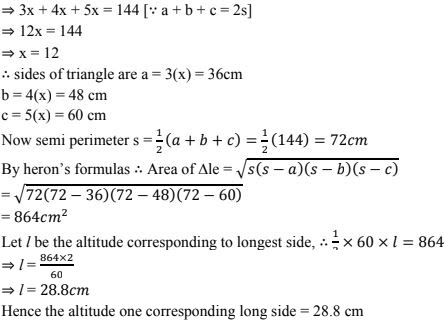10. The perimeter of an isosceles triangle is  42 cm and its base is (3/2) times each of the equal sides. Find the length of each side of the triangle, area of the triangle and the height of the triangle.
Solution
Let 'x' be the measure of each equal sides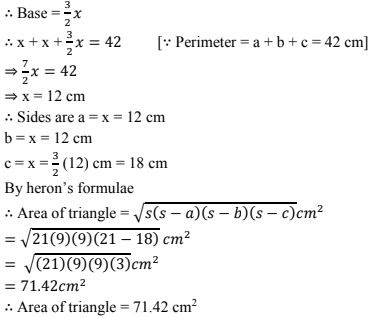11. Find the area of the shaded region in Fig. Below.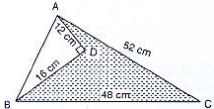Solution
Area of shaded region = Area of ΔABC - Area of ΔADB